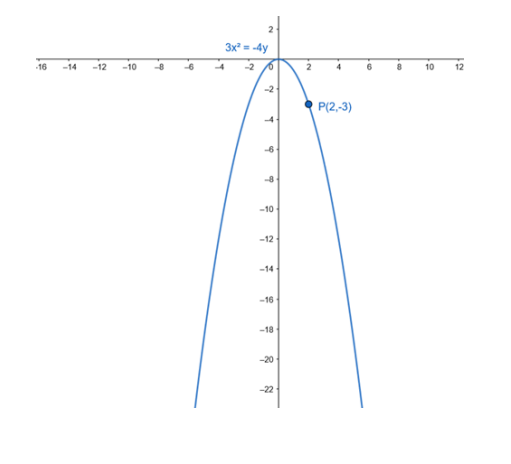Deepak Scored 45->99%ile with Bounce Back Crack Course. You can do it too!

# Find the equation of the parabola, which is symmetric about the y-axis and

Question:

Find the equation of the parabola, which is symmetric about the y-axis and passes through the point P(2, -3).

Solution:

The equation of a parabola with vertex at the origin and symmetric about the y-axis is

$x^{2}=4 a y$

Since point $P(2,-3)$ passes through above parabola we can write,

$2^{2}=4 a(-3)$

$-4=-12 \mathrm{a}$

$\cdot a=-\frac{1}{3}$

Therefore, the equation of a parabola is

$x^{2}=4 \cdot\left(-\frac{1}{3}\right) y$

$x^{2}=-\frac{4}{3} y$

- $3 x^{2}=-4 y$